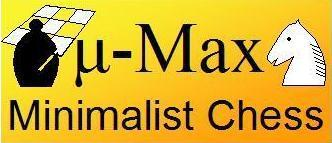This is the source of the smallest micro-Max version. It measures 1180 characters, not counting leading spaces and linefeeds. (But no e.p. capture or castling....) According to the counting rules of the IOCCC its size is even 1088 characters, since they don't count ; { } either!

I could leave out the code line `if(p==4)v+=9*(e>C?!((x^y)&68):-3);`, (that's 33 characters...), but in that case the engine would have severe difficulty finding the mate in KQK. This line awards the King of the side that is ahead by a Queen or more for moving in the same quarter of the board as the enemy King. With transposition tables this evaluation term is not needed, since then the mate is well within the horizon. So you won't see it in the bigger micro-Max.

```#define F(I,S,N) for(I=S;I<N;I++)
#define W(A) while(A)

int V=112,M=136,I=8e3,C=799,X,Y,Q,N,
d[]={-16,-15,-17,0,1,16,0,1,16,15,17,0,14,18,31,33,0,
1,1,3,-1,3,5,9,
7,-1,11,6,8,3,6},
b={6,3,5,7,4,5,3,6};

char n[]=".?+pkltd?*?PKLTD";

D(k,q,l,e,x,n)
int k,q,l,e,x,n;
{
int i=0,j,t,p,u,r,y,m=n>1|q>e?q:e,v,h,z;

N++;
do{
u=b[i];
if(u&k)
{r=p=u&7;
j=d[p+23];
W(r=p>2&r<0?-r:-d[++j])
{y=i;
do
{y+=r;if(y&M)break;
t=b[y];if(t&k|p<3&!(r&7)-!t)break;
v=99*d[t&7|16];
if(v<0)m=I;
if(m>=l)return m;

if(h=n-(y!=x))
{if(p<6)v+=b[i+8]-b[y+8];
if(p==4)v+=9*(e>C?!((x^y)&68):-3);

b[i]=0;b[y]=u;
if(p<3)
{v-=9*(((i-2)&M||b[i-2]!=u)+((i+2)&M||b[i+2]!=u)-1);
if(y+r+1&128){b[y]|=7;v+=C;}
}
v=-D(24-k,-l,-m,z=-e-v,y,h);
v-=v>I/3;
if(x==9){if(v+I&&i==X&y==Y){Q=z;return l;}v=m;}
b[i]=u;b[y]=t;

if(v>m){m=v;if(x&8){X=i;Y=y;}}
}
t+=p<5;if(p<3&&6*k+(y&V)==128)t--;
}W(!t);
}
}
}W(i=i+9&~M);
return m+I?m:-D(24-k,-I,I,0,x,1)/2;
}

main()
{
int k=8,*p,c,d;

F(X,0,8)
{b[X+V]=(b[X]+=16)-8;b[X+16]=18;b[X+96]=9;
F(Y,0,8)b[16*Y+X+8]=(X-4)*(X-4)+(Y-3.5)*(Y-3.5);
}

W(1)
{F(N,0,121)printf(" %c",N&8&&(N+=7)?10:n[b[N]&15]);
p=c;W((*p++=getchar())>10);
if(*c-10){X=*c-16*c+C;Y=c-16*c+C;}else
{d=6;N=0;W(N<1e6)D(k,-I,I,Q,8,d++);}
if(D(k,-I,I,Q,9,2)==I)k^=24;
}
}

```